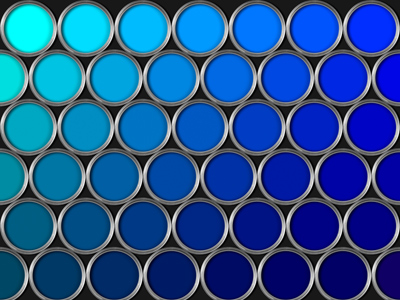What color would you like your room to be?

# Ratio and Proportion

This Math quiz is called 'Ratio and Proportion' and it has been written by teachers to help you if you are studying the subject at middle school. Playing educational quizzes is a fabulous way to learn if you are in the 6th, 7th or 8th grade - aged 11 to 14.

It costs only \$12.50 per month to play this quiz and over 3,500 others that help you with your school work. You can subscribe on the page at Join Us

Ratio is used to indicate how much of one thing there is compared to another. When talking about ratio it is a good idea to use the word PART with it, such as ‘one part juice to 5 parts water’. Then a part might be a cup, a liter, or a jugful. See how well you can work with ratio in this middle school Math quiz.

One of the key things about ratio is that it doesn’t include units, and so can be applied to a variety of situations. Replace the word ‘part’ with whatever unit you want – as long as you are consistent, you will mix things in the right ratio. Ratio is most commonly written using a colon to separate the two (or more) things, such as 1:5. We would read this as ‘1 part to 5 parts’.

Just like fractions, ratios can be canceled down and written in their simplest form, by dividing each number by the Highest Common Factor (HCF).

Another way you may be asked to write a ratio is in the form 1:n. In this case, ask yourself what you need to divide the left-hand side by to get to 1 (it will be itself), and do the same to the right-hand side. This is particularly useful if it is going to be scaled up in the future.

You need to know how to divide a quantity up into a given ratio. These are the trickiest type of ratio questions, but with a little bit of thought they don’t have to be. The key here is to ask yourself how many parts in total, and then work out the value of 1 part. So, if £25 is to be split in the ratio 2:3, there are 5 parts in total, so each part is worth £5. The money would be split £10 to £15.

Question 1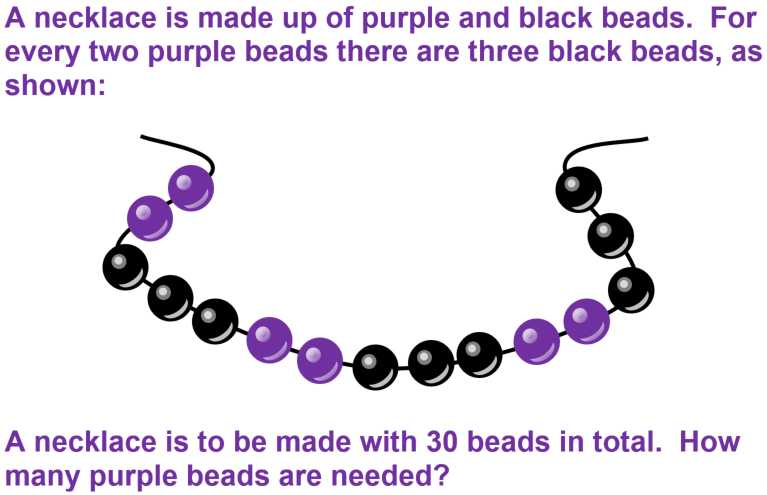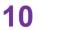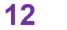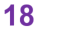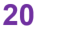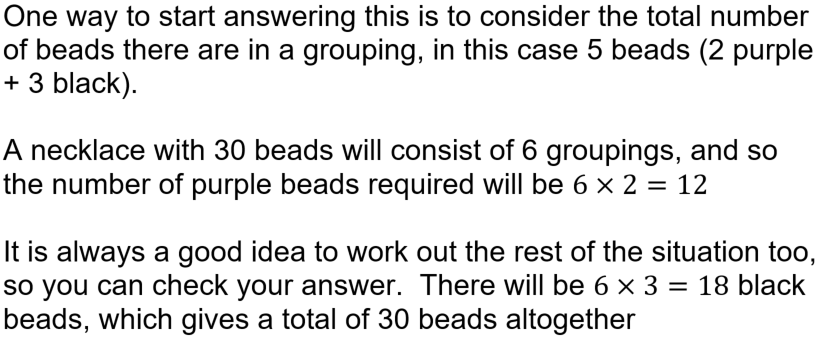Question 2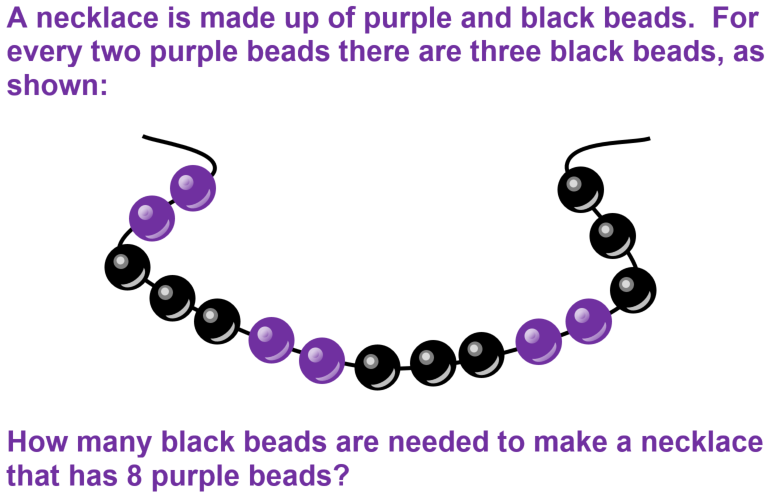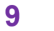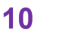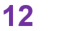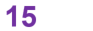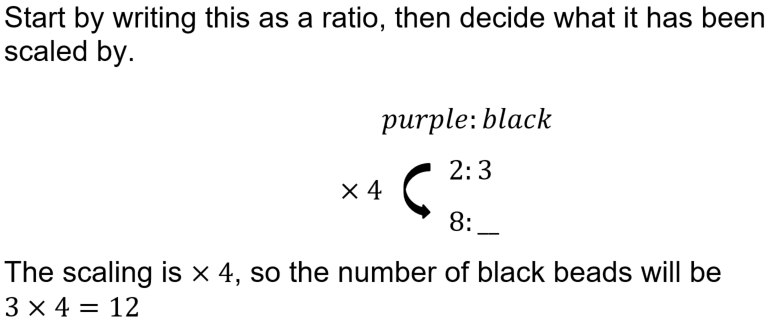Question 3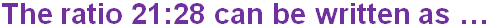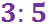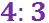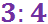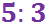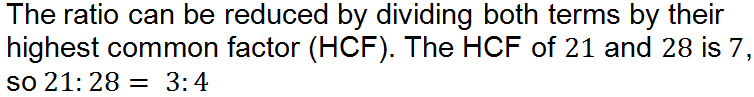Question 4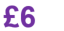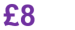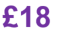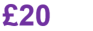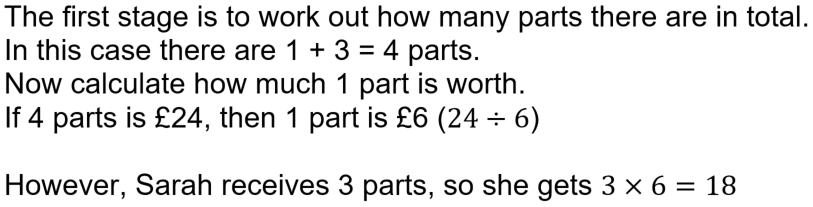Question 5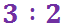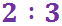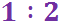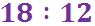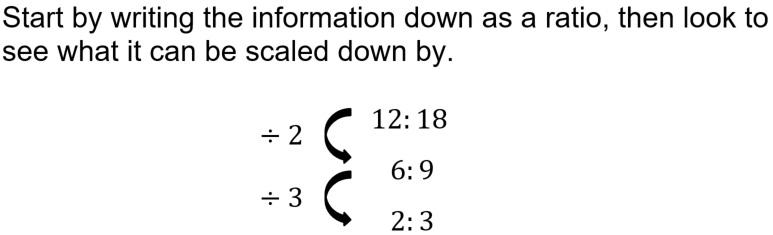Question 6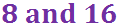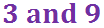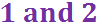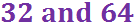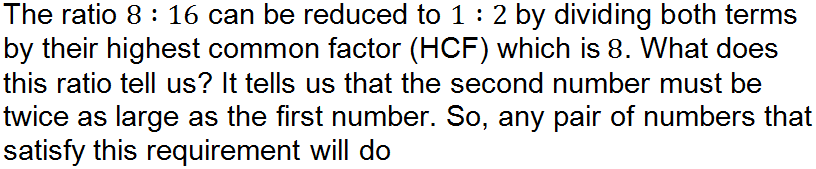Question 7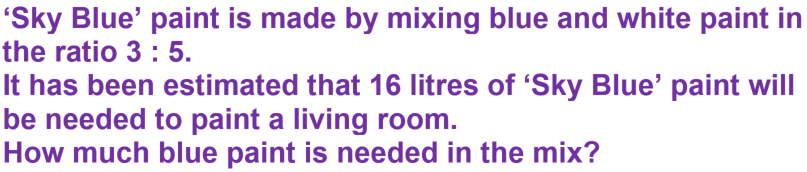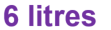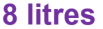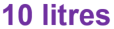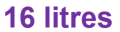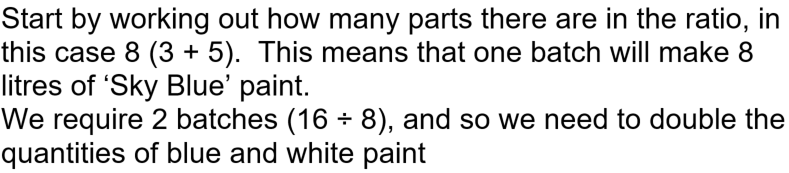Question 8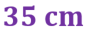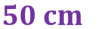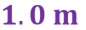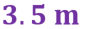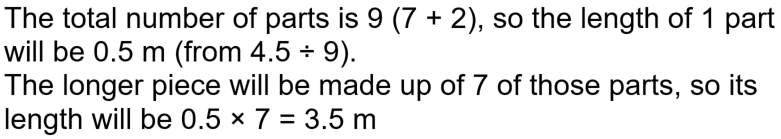Question 9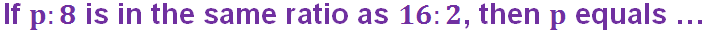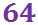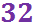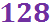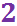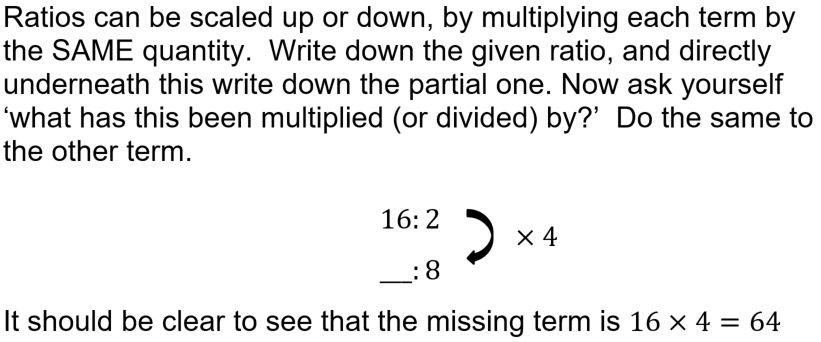Question 10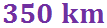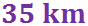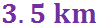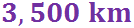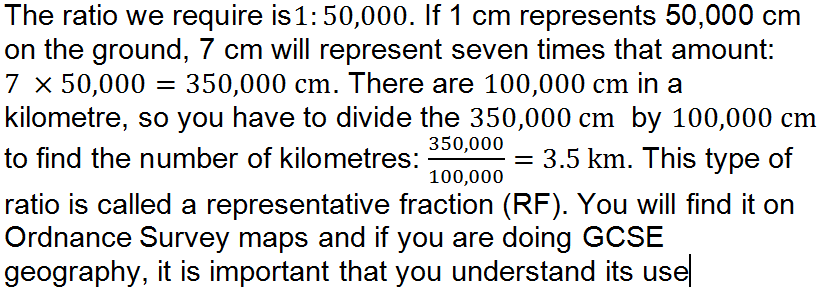Author:  Sally Thompson